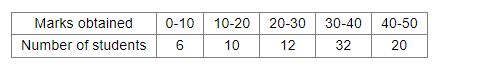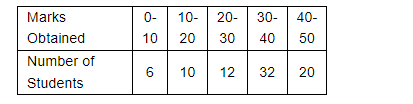# Find the mode of the following distribution of marks obtained by 80 students:`
Question:

Find the mode of the following distribution of marks obtained by 80 students:Solution:

We have the following distributionWe have to find the mode of the above distribution

From the given distribution, we can easily see that the class (20-30) has the maximum frequency i.e. 32.

Lower limit of the modal class $x_{k}=30$

Class interval $h=40-30=10$

Frequency of the modal class $f_{k}=32$

Frequency of the class preceding the modal class $f_{k-1}=12$

Frequency of the class succeeding the modal class $f_{k+1}=20$

Mode $=x_{k}+h\left(\frac{f_{k}-f_{k-1}}{2 f_{k}-f_{k-1}-f_{k+1}}\right)$

$=30+10\left(\frac{32-12}{2 \times 32-12-20}\right)$

$=30+10 \times \frac{20}{32}$

$=36.25$

Therefore the mode value of the given distribution is 36.25